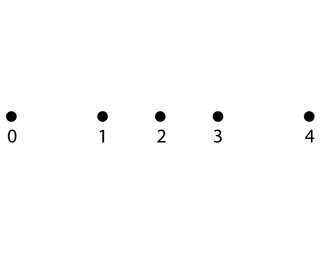# Problem: The shows five points of a motion diagram.Find the average acceleration vectors at points 1, 2, and 3. Draw the completed motion diagram showing velocity vectors and acceleration vectors.

⚠️Our tutors found the solution shown to be helpful for the problem you're searching for. We don't have the exact solution yet.

###### Problem Details

Theshows five points of a motion diagram.

Find the average acceleration vectors at points 1, 2, and 3. Draw the completed motion diagram showing velocity vectors and acceleration vectors.